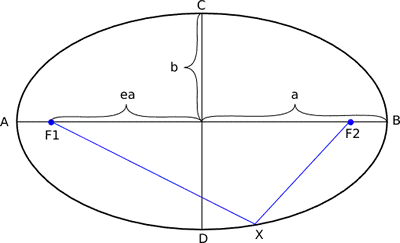×

# Ellipse Circumference CalculatorSemi-major axis length (a):: Semi-minor axis length (b)::
Ellipse Circumference ≈
20.957036908

We notice you're using an adblocker. We made hundreds of free online tools and calculators – it costs a lot.
If you like our tools please keep us running by whitelisting this site in your ad blocker. We’re serving quality, related ads only. Or you can make a donation to support us. Thank you!

I've whitelisted miniwebtool.

## About Ellipse Circumference Calculator

The online Ellipse Circumference Calculator is used to calculate the approximate circumference of an ellipse.

## Ellipse

In geometry, an ellipse is a regular oval shape, traced by a point moving in a plane so that the sum of its distances from two other points (the foci) is constant, or resulting when a cone is cut by an oblique plane that does not intersect the base.

## Formula

There is no simple formula with high accuracy for calculating circumference of an ellipse. The following is the approximate calculation formula for the circumference of an ellipse used in this calculator:Where:
a = semi-major axis length of an ellipse
b = semi-minor axis length of an ellipse
π = 3.141592654

## Related

Watch Videos:

©2019 Miniwebtool | Terms and Disclaimer | Privacy Policy | Contact Us